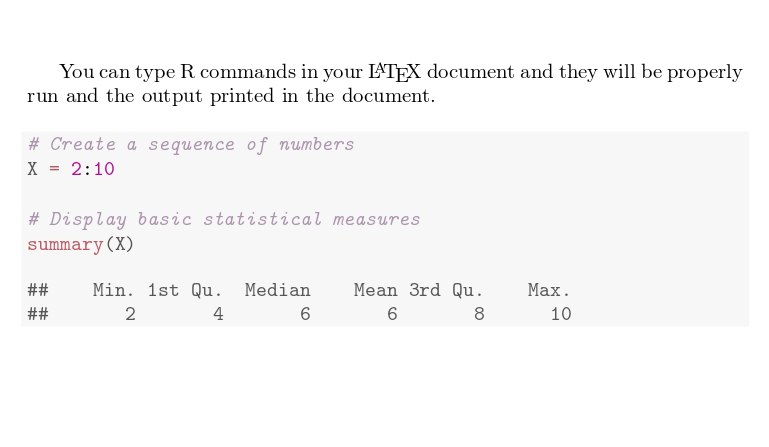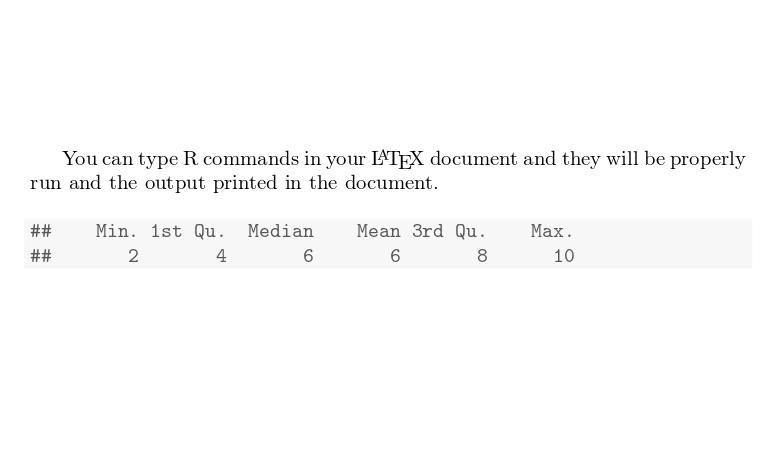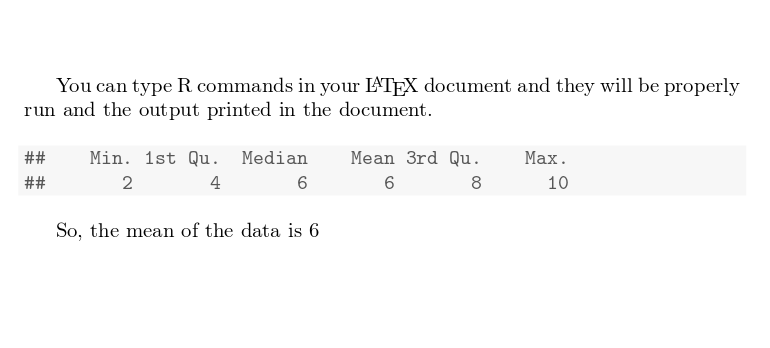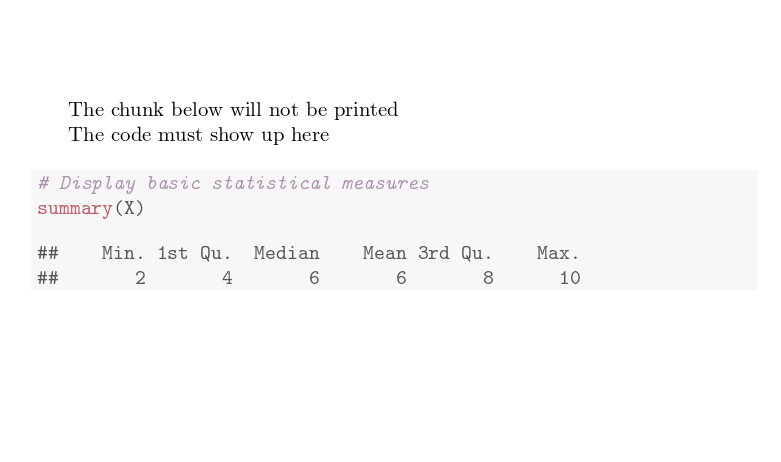Showing first {{hits.length}} results of {{hits_total}} for {{searchQueryText}}{{hits.length}} results for {{searchQueryText}}

No Search Results

As noted on Wikipedia, Knitr is an engine for dynamic report generation with R, a statistics-oriented programming language. This article explains how to add R code to your LaTeX document to generate a dynamic output. In a standard LaTeX distribution you must have R set up in your operating system and run some special commands to compile it. Overleaf can save you the trouble, knitr works out of the box. Contents 1 Introduction 2 Chunks of code 3 Inline commands 4 Plots 5 External R scripts 6 Reference guide 7 Further reading Introduction Documents that contain R code must be saved with the extension .Rtex, otherwise the code won't work. Let's see an example: \documentclass{article} \usepackage[utf8]{inputenc} \usepackage[english]{babel} \begin{document} You can type R commands in your \LaTeX{} document and they will be properly run and the output printed in the document. <<>>= # Create a sequence of numbers X = 2:10 # Display basic statistical measures summary(X) @ \end{document}As you see, the text in between the characters <<>>= and @ is R code, this code and its output is printed in a listing-like format. This chunk of code can take some extra parameters to customize the dynamic output. See the next section.   Open an example of the knitr package in Overleaf Chunks of code A code block as the one presented in the previous section is usually called a chunk. You can set some extra options in knitr chunks. See the example below: You can type R commands in your \LaTeX{} document and they will be properly run and the output printed in the document. <>= # Create a sequence of numbers X = 2:10 # Display basic statistical measures summary(X) @There are three additional options passed inside << and >>. echo=FALSE This hides the code and only prints the output generated by R. cache=TRUE If cache is set to true the chunk is not run, only the objects generated by it. This saves time if the data in that chunk haven't changed. Note that the cache=TRUE option is not currently supported in Overleaf, but it should work locally. See the reference guide for more options.   Open an example of the knitr package in Overleaf Inline commands It is possible to access objects generated in a chunk and print them in-line. You can type R commands in your \LaTeX{} document and they will be properly run and the output printed in the document. <>= # Create a sequence of numbers X = 2:10 # Display basic statistical measures summary(X) @ So, the mean of the data is $\Sexpr{mean(X)}$The command \Sexpr{mean(X)} prints the output returned by the R code mean(X). Inside the braces any R command can be passed.   Open an example of the knitr package in Overleaf Plots Plots can also be added to a knitr document. See the next example <>= xdata = read.csv(file="data.txt", head=TRUE,sep=" ") hist(xdata\$data, main="Overleaf histogram", xlab="Data") @ The figure \ref{fig:plot1} is simple histogram.This histogram uses data stored in "data.txt", saved in the current working directory. A few figure-related options are passed to the chunk. plot1 This is the label used to reference the plot. The prefix "fig:" is mandatory. You can see in the example that the figure is referenced with \ref{fig:plot1}. fig.pos="t" Positioning parameter. This is the same used in the figure environment. fig.height=4, fig.width=4 Figure width and height fig.cap="First plot" Caption for the figure.   Open an example of the knitr package in Overleaf External R scripts You can import parts of an external R script into a knitr document. This is very helpful since is fairly common to write and debug the script in an external program prior to including it in your document. Let's see an example: suppose you need the next file to be included in your LaTeX document: ## ---- myrcode1 # Create a sequence of numbers X = 2:10 ## ---- myrcode2 # Display basic statistical measures summary(X) Notice the lines ## ---- myrcode1 These mark the beginning of a chunk of code and are mandatory if you want to use this script in your document. The chunk below will not be printed <>= read_chunk("mycode.R") @ The code must show up here <>= @The first chunk is not printed, is only used to import the script with the command read_chunk("mycoder.R"), that's why the option echo=FALSE is set. Also, scripts mus not be cached. Once the script is imported, you can print a chunk using the label you set after ## ----. In this case it's myrcode2   Open an example of the knitr package in Overleaf Reference guide Some chunk options results. Changes the behaviour of the results generated by the R code, possible values are markup Use LaTeX for format the output. asis Prints raw results from R. hold Holds the output results and to push them at the end of the chunk. hide Hide results. echo. Whether to include the R source code. Can take other parameters, echo=2:3 prints only the second and third lines; echo=-2:-3 excludes the second and third lines only. cache. Whether to cache the code chunk. Possible values are TRUE and FALSE highlight. Whether to highlight the source code. Possible values are TRUE and FALSE background. Background colour of the chunk, rgb and HTML formats can be used, the default value is "#F7F7F7". Further reading For more information see Code Highlighting with minted Using colours in LaTeX plots in LaTeX Inserting Images Tables Positioning images and tables TikZ package Mathematical expressions the knitr web page the knitr package manual